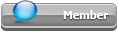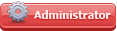## Featured Articles

Check out the latest featured articles.## File Library0# Pressure Drop For Vertical Piping

pressure drop vertical piping height friction pressure gas

3 replies to this topic
|

### #1zamakaze

zamakaze

Junior Member

•• Members
•• 12 posts

Posted 01 June 2021 - 02:59 PM

hlo,

im trying to calculate the pr. drop in vertical piping for gas.

i have a vertical section where gas goes down in elevation 5 ft

the pressure at starting point is 85 psig

since there is fall of 5 ft i assume pr. gain can be calculate as = rho x g x h2-h1 where h2 is 5ft , h1 is 0 ft

for frictional drop can we just use the darcy eqn for vertical or there are some other considerations??

attachd sktch

### #2latexman

latexman

Gold Member

••• 1,321 posts

Posted 01 June 2021 - 03:30 PM

That looks right.  It's the Bernoulli equation with friction included.   Don't forget the fittings (2 elbows).

### #3breizh

breizh

Gold Member

••• 5,379 posts

Posted 01 June 2021 - 07:05 PM

hi,

The head loss due to the vertical part (altimetry) is very minimum ,negligable .  Bernoulli equation to apply.

Don't forget the elbows in your calculation .

Good luck

Breizh

### #4fallah

fallah

Gold Member

•• ChE Plus Subscriber
•• 4,825 posts

Posted 01 June 2021 - 10:41 PM

im trying to calculate the pr. drop in vertical piping for gas.

i have a vertical section where gas goes down in elevation 5 ft

the pressure at starting point is 85 psig

since there is fall of 5 ft i assume pr. gain can be calculate as = rho x g x h2-h1 where h2 is 5ft , h1 is 0 ft

for frictional drop can we just use the darcy eqn for vertical or there are some other considerations??

attachd sktch

Hi,

For gaseous fluid there is no significant difference in pressure drop calculation for vertical and horizontal lines...

### Similar Topics